Ex.3.7 (Optional)

Chapter 3 Class 10 Pair of Linear Equations in Two Variables
Serial order wise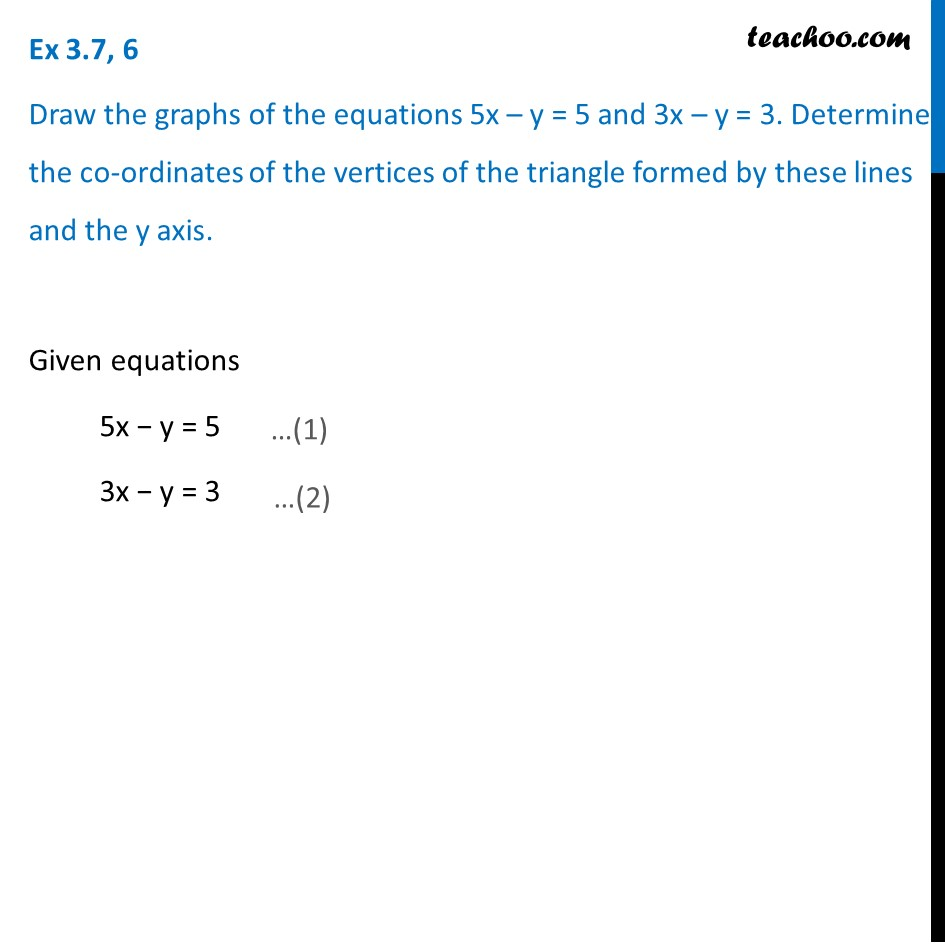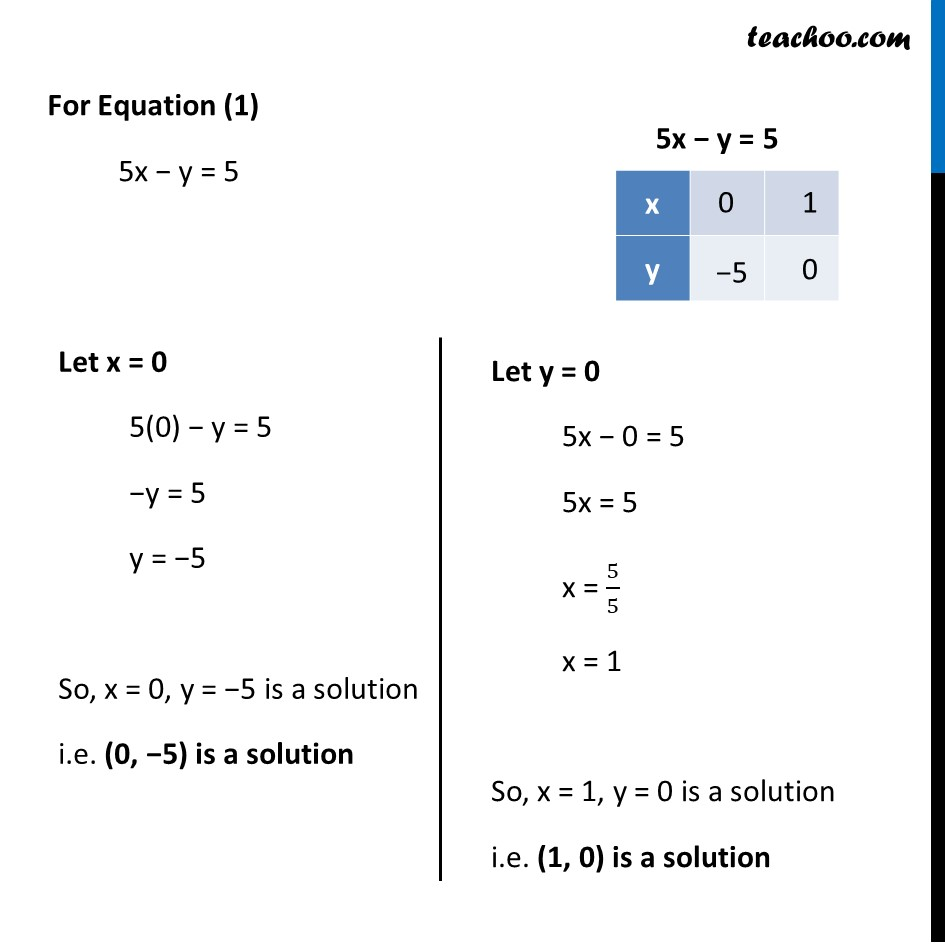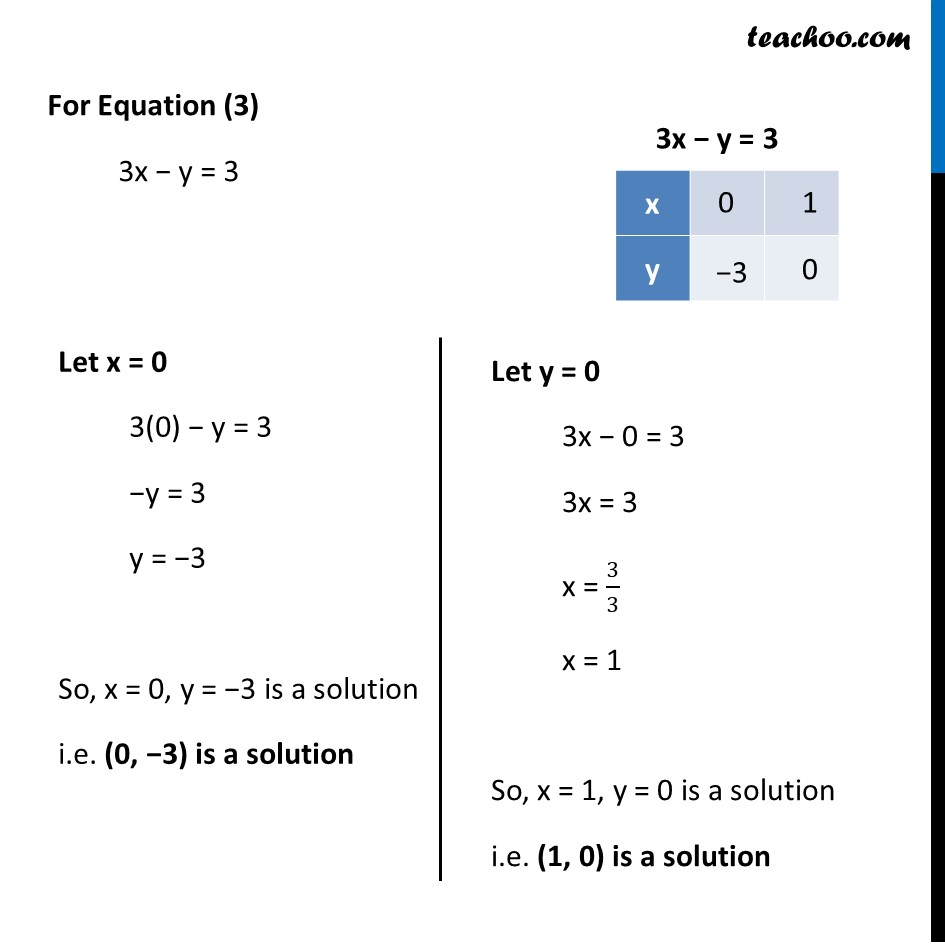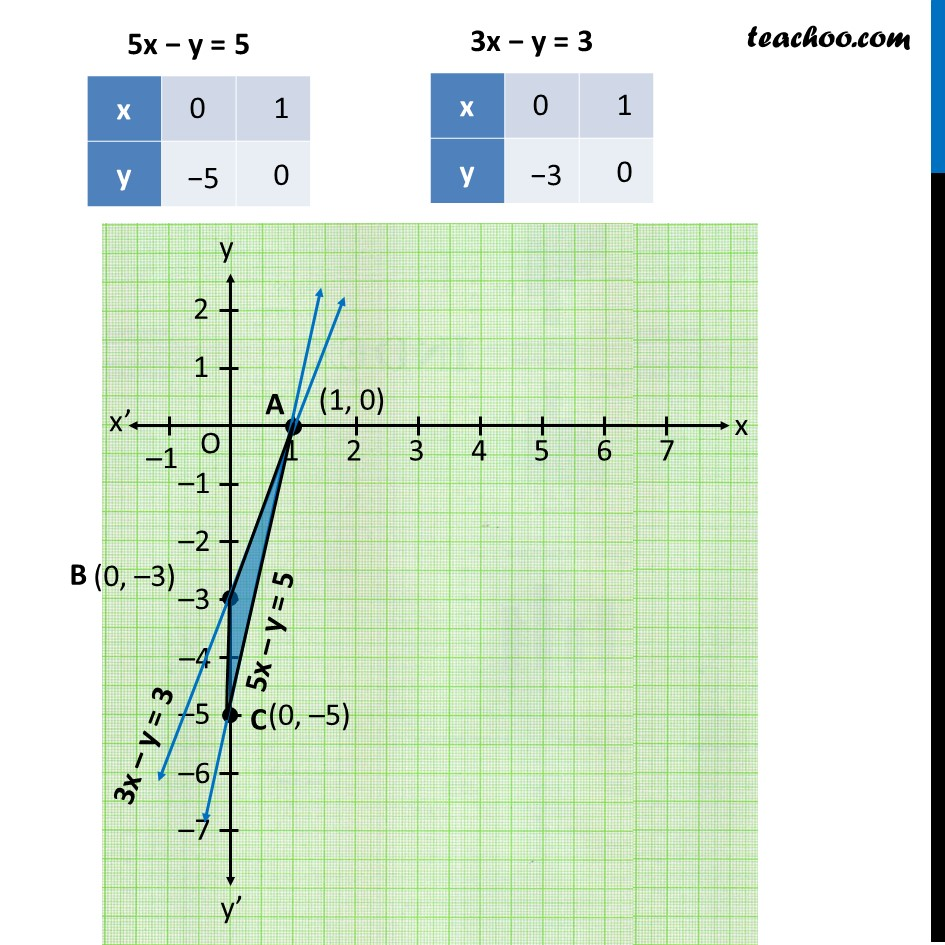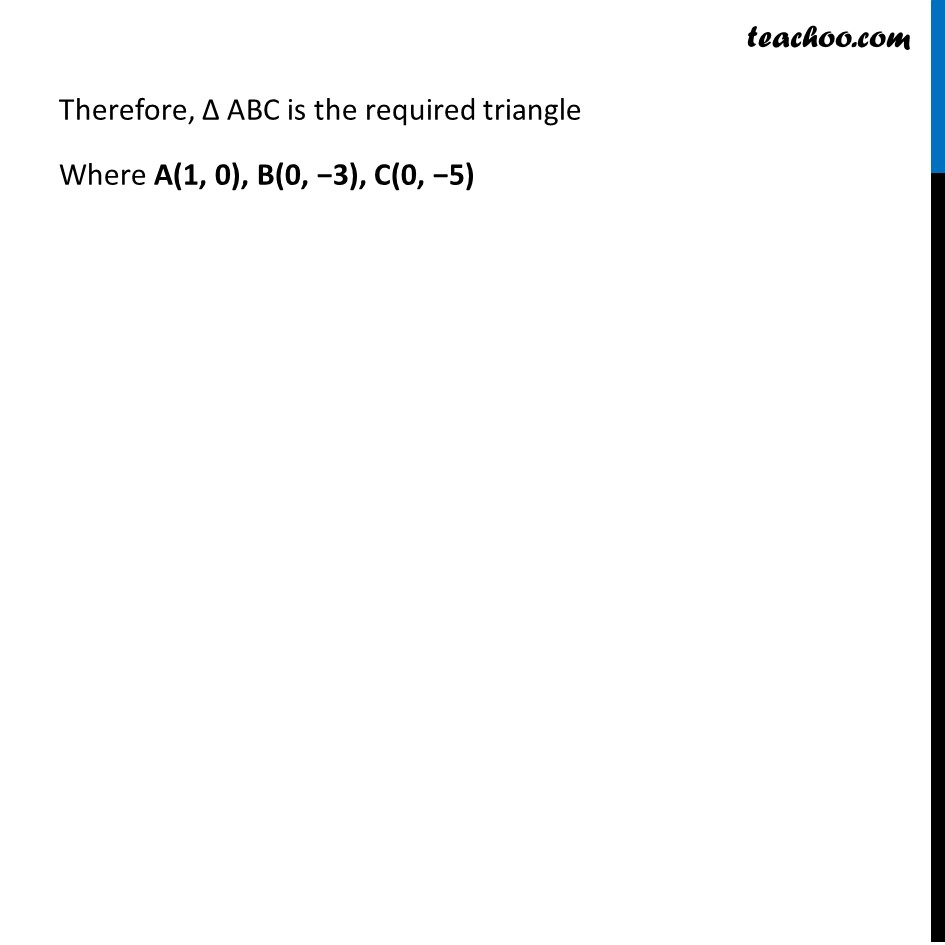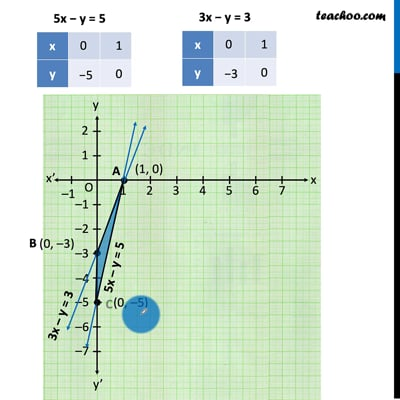This video is only available for Teachoo black users

Maths Crash Course - Live lectures + all videos + Real time Doubt solving!

### Transcript

Ex 3.7, 6 Draw the graphs of the equations 5x – y = 5 and 3x – y = 3. Determine the co-ordinates of the vertices of the triangle formed by these lines and the y axis. Given equations 5x − y = 5 3x − y = 3 For Equation (1) 5x − y = 5 Let x = 0 5(0) − y = 5 −y = 5 y = −5 So, x = 0, y = −5 is a solution i.e. (0, −5) is a solution Let y = 0 5x − 0 = 5 5x = 5 x = 5/5 x = 1 So, x = 1, y = 0 is a solution i.e. (1, 0) is a solution For Equation (3) 3x − y = 3 Let x = 0 3(0) − y = 3 −y = 3 y = −3 So, x = 0, y = −3 is a solution i.e. (0, −3) is a solution Let y = 0 3x − 0 = 3 3x = 3 x = 3/3 x = 1 So, x = 1, y = 0 is a solution i.e. (1, 0) is a solution Therefore, Δ ABC is the required triangle Where A(1, 0), B(0, −3), C(0, −5)